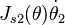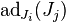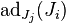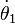# Modern Robotics Errata

Errata below are for the hardcover book published by Cambridge University Press and the preprint version of the book posted on May 3, 2017. Thanks to everyone who provided corrections to earlier versions!

## Significant corrections

### Chapter 5

• Equation (5.7): The second term on the right-hand side of the equation should be$J_{s2}(\theta) \dot{\theta}_2$.

### Chapter 6

• Chapter 6.3, first line after Eq (6.7): the matrices$T_{sd}^{-1} \dot{T}_{sd}$ and$\dot{T}_{sd} T_{sd}^{-1}$ are referred to as twists, but these are the se(3) matrix representations of the twists.

### Chapter 8

• Figure 8.5 says the volume of the rectangular parallelepiped is abc but it should be hlw.
• Exercise 8.6(a): The expression$\text{ad}_{J_i}(J_j)$ has the indices switched; the correct expression is$\text{ad}_{J_j}(J_i)$.

### Chapter 11

• In the displayed equation after Equation (11.18), the vector Xe(t) is a six-vector. The bottom three elements are written correctly, but the top three elements, an angular velocity, are written instead in their 3x3 so(3) form.

## Minor typos, etc., no danger of misunderstanding

### Chapter 1

• (online version only) Description of Chapter 6: "jont positions" should be "joint positions."

### Chapter 2

• Chapter 2.2.2, Example 2.3: "Substituting" is misspelled.

### Chapter 6

• (online version only) Figure 6.7: θ0 should be θ0 (five times) and θ1 should be θ1 (one time).

### Chapter 7

• Just below caption for Figure 7.8: There is an extraneous dot.

### Chapter 8

• (online version only) Chapter 8.1.1, last paragraph:$\dot{\theta}_1$ is improperly typeset.

## A partial list of errata contributors

Thanks to the following people who provided corrections, starting from the preliminary version of the book posted in October, 2016:

H. Andy Nam, Eric Lee, Yuchen Rao, Chainatee Tanakulrongson, Mengjiao Hong, Kevin Cheng, Jens Lundell, Elton Cheng, Michael Young, Jarvis Schultz, Logan Springgate, Sofya Akhmametyeva, Aykut Onol, Josh Holcomb, Yue Chen, Mark Shi, AJ Ibraheem, Yalun Wen, Seongjae Jeong